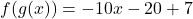## If f(x) = 5x + 7 and g(x) = -2x – 4 Find f(g(x)) = -10x + [?] Enter​

Question

If f(x) = 5x + 7 and g(x) = -2x – 4 Find f(g(x)) = -10x + [?] Enter​

in progress 0
7 months 2021-08-07T16:09:29+00:00 1 Answers 2 views 0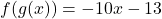Step-by-step explanation:

Given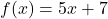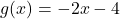Required

Find f(g(x))

This is calculated as:Substitute g(x) for x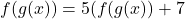Substitute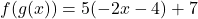Open bracket